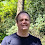## Overview

The order you add double values can give you different results. This gets worse as the sum approaches 0 as the error is large compared with the result.

Something I found interesting recently is seeing how many possible results you can get depending on the order you sum the values.

## Looking at variations on the sum

In the following code, the program creates random numbers around zero, adding a value to the list which ensures the sum is almost zero. It lists the different sums it finds.
```List<Double> doubles = new ArrayList<Double>();
Random rand = new Random();
double sum0 = 0;
for (int i = 0; i < 1000; i++) {
sum0 += doubles.get(doubles.size() - 1);
}

SortedSet<Double> sums = new TreeSet();
for (int i = 0; i < 10 * 1000 * 1000; i++) {
Collections.shuffle(doubles, rand);
double sum = 0;
for (double d : doubles)
sum += d;
System.out.println(sum);
}
System.out.printf("Found %,d different sums from %g to %g%n", sums.size(), sums.first(), sums.last());
```

prints

```-1.0891287871572786E-13
5.184741524999481E-14
-1.0469403122215226E-13
-1.1235457009206584E-13
3.985700658404312E-14
... many snipped ...
-1.042499420123022E-13
7.37188088351104E-14
-1.1379786002407855E-13
-1.084687895058778E-13
-1.0591527654923993E-13
Found 1,411 different sums from -1.39000e-13 to 7.37188e-14
```
For an array of 100, it found 156 possible sums. For 10,000 values you can get
```Found 12,701 different sums from -8.01137e-13 to 1.59517e-12
```

To get an exact answer you can use BigDecimal. This will give you same result regardless of order.

```BigDecimal bd = BigDecimal.ZERO;
for (double d : doubles)
Collections.shuffle(doubles, rand);
BigDecimal bd2 = BigDecimal.ZERO;
for (double d : doubles)
if (!bd.equals(bd2))
throw new AssertionError();
System.out.println("The actual sum is exactly "+bd);
```
and prints something like
```The actual sum is exactly 4.77395900588817312382161617279052734375E-15
```

## Is an increasing or decreasing sequence better

I would think that for positive number, an increasing sequence would produce a more accurate sum. In this case, there is both negative and positive values and sorting by the absolute value doesn't help much.
```List doubles = new ArrayList();
for (int s = 0; s < 20; s++) {
Random rand = new Random(s);
double sum0 = 0;
for (int i = 0; i < 1000; i++) {
sum0 += doubles.get(doubles.size() - 1);
}

BigDecimal actualSum = BigDecimal.ZERO;
for (double d : doubles)
System.out.printf("%d: Actual sum %s, ", s, "" + actualSum.doubleValue());

SortedSet increasing = new TreeSet(new Comparator() {
@Override
public int compare(Double o1, Double o2) {
return Double.compare(Math.abs(o1), Math.abs(o2));
}
});
if (increasing.size() != doubles.size()) throw new AssertionError();

double increasingSum = 0;
for (double d : increasing)
increasingSum += d;
System.out.print("increasingSum= " + increasingSum+", ");

SortedSet decreasing = new TreeSet(new Comparator() {
@Override
public int compare(Double o1, Double o2) {
return -Double.compare(Math.abs(o1), Math.abs(o2));
}
});
if (decreasing.size() != doubles.size()) throw new AssertionError();

double decreasingSum = 0;
for (double d : decreasing)
decreasingSum += d;
System.out.println("decreasingSum= " + decreasingSum);
}
```
prints
```0: Actual sum 1.5432100042289676E-14, increasingSum= 2.8421709430404007E-14, decreasingSum= 2.9531932455029164E-14
1: Actual sum -9.880984919163893E-15, increasingSum= 3.552713678800501E-14, decreasingSum= 1.5432100042289676E-14
2: Actual sum -2.4424906541753444E-15, increasingSum= -2.8421709430404007E-14, decreasingSum= -1.2989609388114332E-14
3: Actual sum 3.9968028886505635E-15, increasingSum= -7.105427357601002E-15, decreasingSum= -1.587618925213974E-14
4: Actual sum 7.105427357601002E-15, increasingSum= 7.105427357601002E-15, decreasingSum= 6.328271240363392E-14
5: Actual sum 2.142730437526552E-14, increasingSum= -4.263256414560601E-14, decreasingSum= -2.3314683517128287E-14
6: Actual sum 1.532107773982716E-14, increasingSum= 1.4210854715202004E-14, decreasingSum= 2.375877272697835E-14
7: Actual sum 1.7763568394002505E-15, increasingSum= 3.552713678800501E-15, decreasingSum= 2.4424906541753444E-15
8: Actual sum -8.881784197001252E-16, increasingSum= 3.197442310920451E-14, decreasingSum= 9.2148511043888E-15
9: Actual sum 3.597122599785507E-14, increasingSum= -5.3290705182007514E-14, decreasingSum= -4.6851411639181606E-14
10: Actual sum 3.83026943495679E-14, increasingSum= -1.1013412404281553E-13, decreasingSum= -7.582823258189819E-14
11: Actual sum 3.8413716652030416E-14, increasingSum= 3.197442310920451E-14, decreasingSum= 4.107825191113079E-15
12: Actual sum 2.786659791809143E-14, increasingSum= 1.7763568394002505E-14, decreasingSum= -6.206146707654625E-14
13: Actual sum 2.631228568361621E-14, increasingSum= -1.0658141036401503E-14, decreasingSum= 1.6986412276764895E-14
14: Actual sum 4.030109579389318E-14, increasingSum= 7.460698725481052E-14, decreasingSum= 2.3647750424515834E-14
15: Actual sum 3.175237850427948E-14, increasingSum= -1.0658141036401503E-14, decreasingSum= -6.050715484207103E-14
16: Actual sum 2.4091839634365897E-14, increasingSum= -6.750155989720952E-14, decreasingSum= -1.084687895058778E-13
17: Actual sum 1.709743457922741E-14, increasingSum= -2.0961010704922955E-13, decreasingSum= -1.8551826741486366E-13
18: Actual sum 2.930988785010413E-14, increasingSum= -9.592326932761353E-14, decreasingSum= -1.341149413747189E-13
19: Actual sum 2.3092638912203256E-14, increasingSum= -1.0658141036401503E-14, decreasingSum= -1.0058620603103918E-13
```

1.It may be worth mentioning that the exact sum can also be computed using floating-point math and without re-ordering the data: http://dl.acm.org/citation.cfm?id=1824815.

(Here, "exact" means "the nearest valid float/double").

2.@aix, Thank you for the link.

3.This effect rellay doesn't have anything to do with the numbers being close to zero. The rule of thumb is to sort the numbers by their absolute value prior to summing up in order to minimize the error. would be interesting how close you get to the exact result using this approach.

4.@Axel, A good suggestion. I have added a section at the end to compare summing increasing and decreasing absolute value collections.